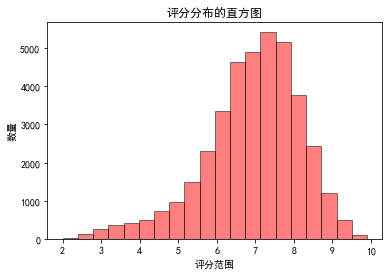## 数据绘图工具之Matplotlib

一.安装：绘图和可视化

pip install matplotlib

我们已经下好了anaconda 包含了绘图工具包 直接导入即可

import matplotlib.pyplotlib as plt

二.# 第一种图形 可视化绘图：折线图

```# 上面由于是中文需要解决乱码的问题

b = [2,5,4,8,9,7,10]

plt.plot(b)
# 设置标题
plt.rcParams['font.sans-serif'] = ['SimHei']
plt.rcParams['axes.unicode_minus'] = False
plt.title('title经济发展趋势图',fontsize=20,color='red')

# 设置 x y 轴的名称
plt.xlabel('x轴',fontsize=20)
plt.ylabel('y轴',fontsize=20)

# 调节图表大小

plt.show()```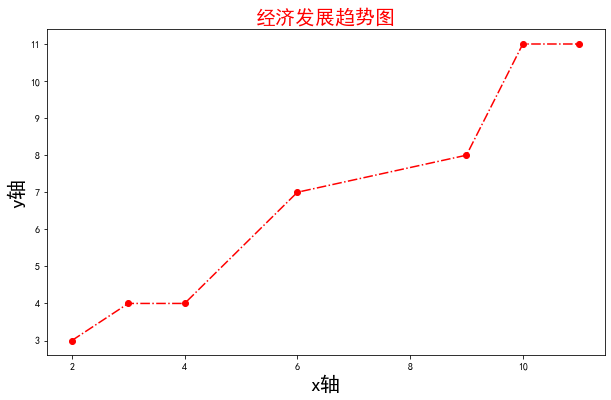(2)第二种 绘制每个国家地区电影数量的柱状图

```#(1）读取数据
mv```
```# (2)每个地区有分区 进行分主 然后进行统计数量 size（）
movies = mv.groupby('产地').size().sort_values(ascending=False)
movies```
```# (3)获取x, y 轴的值
x = movies.index
x

# 获取 y轴的值
y = movies.values
y```
```(4)# 绘制柱状图 bar
# plt.bar(x,y,color='blue')
#  plt.show(）显示图型

# 调节图表大小
plt.figure(figsize=(20,6))  # 注意是画图之前

plt.bar(x,y,color='blue')

# 标题
plt.title('每个国家的或地区的电影数量',fontsize=30,color='k')  #　没有位置固定

# x 轴的字体的的大小 和 旋转角度
plt.xticks(rotation=45,fontsize=15)   # ticks 滴答点  参数 旋转 大小

# x 轴的设置 命名和大小 颜色
plt.xlabel('产地',fontsize=20,color='red')

# y 轴的设置
plt.ylabel('电影数量',fontsize=20,color='red',rotation=90)
# 显示柱状图上的数量
for a,b in zip(x,y,):
plt.text(a,b+200,b,horizontalalignment='center',fontsize=15,color='red')

# 保存图片 到指定的路径
plt.savefig('./电影柱状图.png')
plt.show()```

图形展示：(5)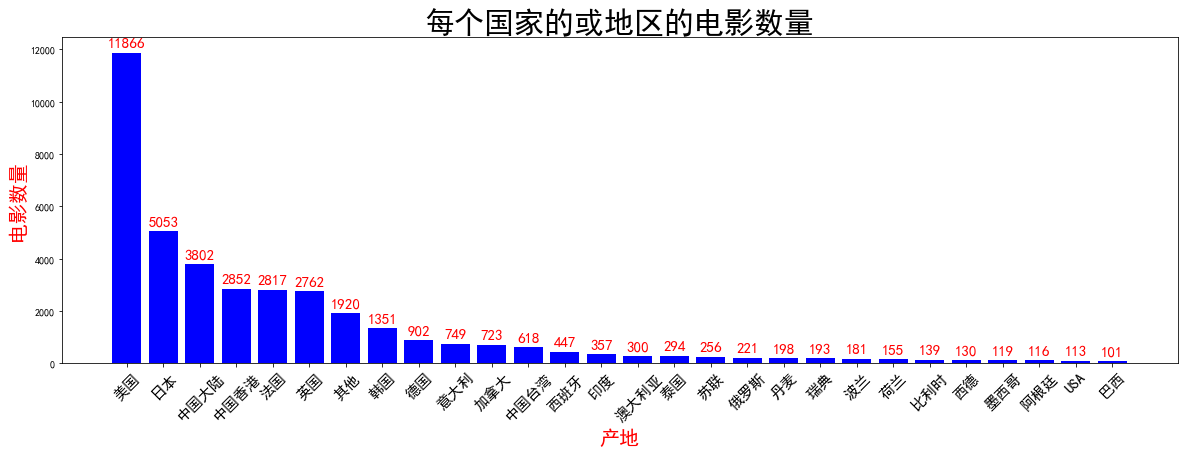（2.1）# 2 根据数据绘制每年电影上映数量的曲线图

`# (1) 按照年代将读取的mv 电影进行进行分组`
```res = mv.groupby(['年代']).size().sort_index()  # 这个可以进行排序
# mv['年代'].value_counts().sort_values()
res = res[:-2]  # 删除 最后两个数
res```

(2)#  设置x z轴的值 和 y 轴的值

```x = res.index
x
# 设置y 轴
y = res.values
y```

(3)绘图

```# 大小是在绘图前
plt.figure(figsize=(10,6))
plt.plot(x,y)
# x 轴的设置
plt.xlabel('年代',fontsize=20,color='k')
plt.ylabel('数量',fontsize=20,color='b')

# x 轴 字体的设置
plt.xticks(fontsize=20,color='r')
plt.show()```

（4）图展示：最后的数据未完成统计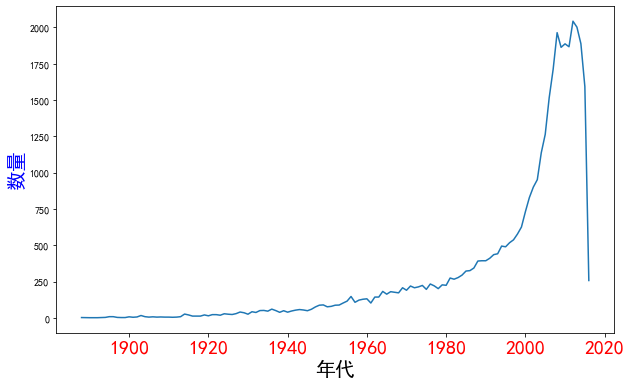# 第三 饼图的设计
pd.cut?

(1)

```# 获取电影时长
time_res = mv['时长']
time_res```

(2)

```# 分等份
res = pd.cut(time_res,[0,60,90,120,140,1000])  # time_res 是带分割的源数据  后面是份额区间
res# 分等份
res = pd.cut(time_res,[0,60,90,120,140,1000])  # time_res 是带分割的源数据  后面是份额区间
res```

(3)

```# 统计区间的电影数量
res = res.value_counts()
res
x = res.index
x
y = res.values
y```

(4)

```# plt.pie(y,labels= x)
plt.title('电影时长分步图',fontsize=20)
patchs, l_text, p_text = plt.pie(y, labels=x, autopct='%0.2f%%',)  # patchs 打补丁
for p in p_text:  # p_text 代表内部的内容    %0.2f%%  0.2 表示保留两位浮点数  后面的% 是在区域内文本值后加%
p.set_size(15)
p.set_color('white')
for l in l_text:
l.set_size(13)
l.set_color('r')

plt.show()```

图形展示：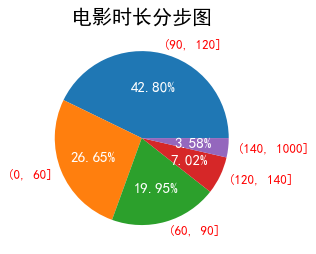# 4 直方图

（1）

```x = mv['评分']
x```

（2）

```# plt.bar(x,y,color='blue')
plt.hist(mv['评分'],bins=20, edgecolor='black',alpha=0.5, facecolor='red')
plt.title('评分分布的直方图')
plt.xlabel('评分范围')
plt.ylabel('数量')
# for a,b in zip(x,y):
#     plt.text(a,b+200,b,horizontalalignment='center',fontsize=15,color='red')

plt.show()```

评分图不是想做成的样子